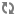Creating Custom Builder Components# Creating UDFs

User Defined Functions (UDFs) allow you to do simple transformations on event streams. This is used in the Projection processor.

1. Create a UDF by implement the following interface:
```public interface UDF<O, I> {
O evaluate(I i);
}
```

Where:

• I – Is the input type.

• O – Is the output type.

• The evaluate method is invoked with the corresponding field value for each event in the stream.

2. For functions that accept two or more parameters, the there are corresponding UDF interfaces (UDF2 to UDF7).
```public interface UDF2<O, I1, I2> {
O evaluate(I1 input1, I2 input2);
}
```

Example 1

The ConvertToTimestampLong UDF is a good example of a new UDF implementation.

Example 2

In this example, you to concatenate the values of two fields of an event. To do this, define a MyConcat function by implementing the UDF2 interface as shown below

```pubic class MyConcat implements UDF2<String, String, String> {
public String evaluate(String s1, String s2) {
return s1.concat(s2);
}
}
```